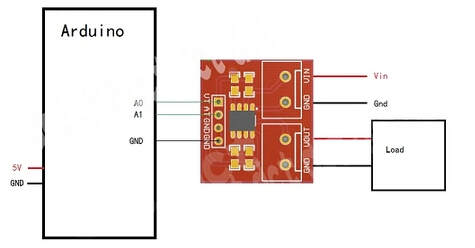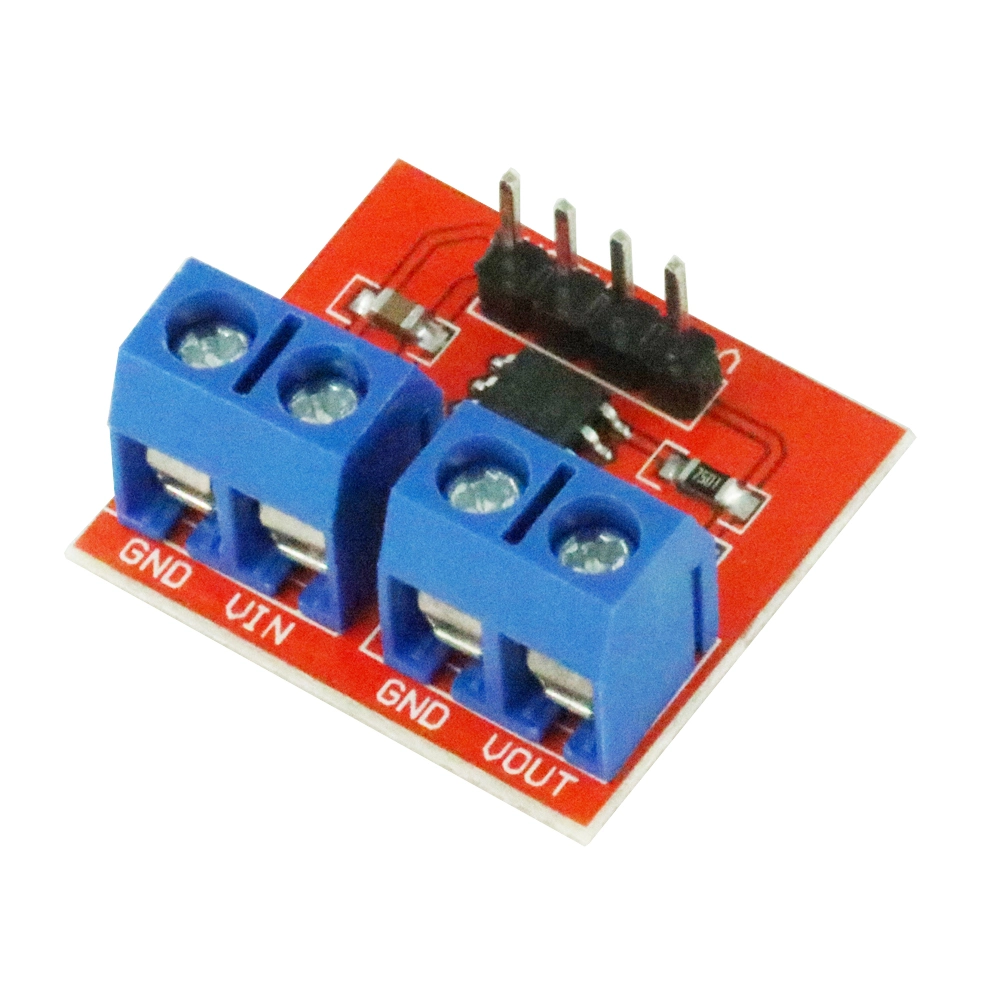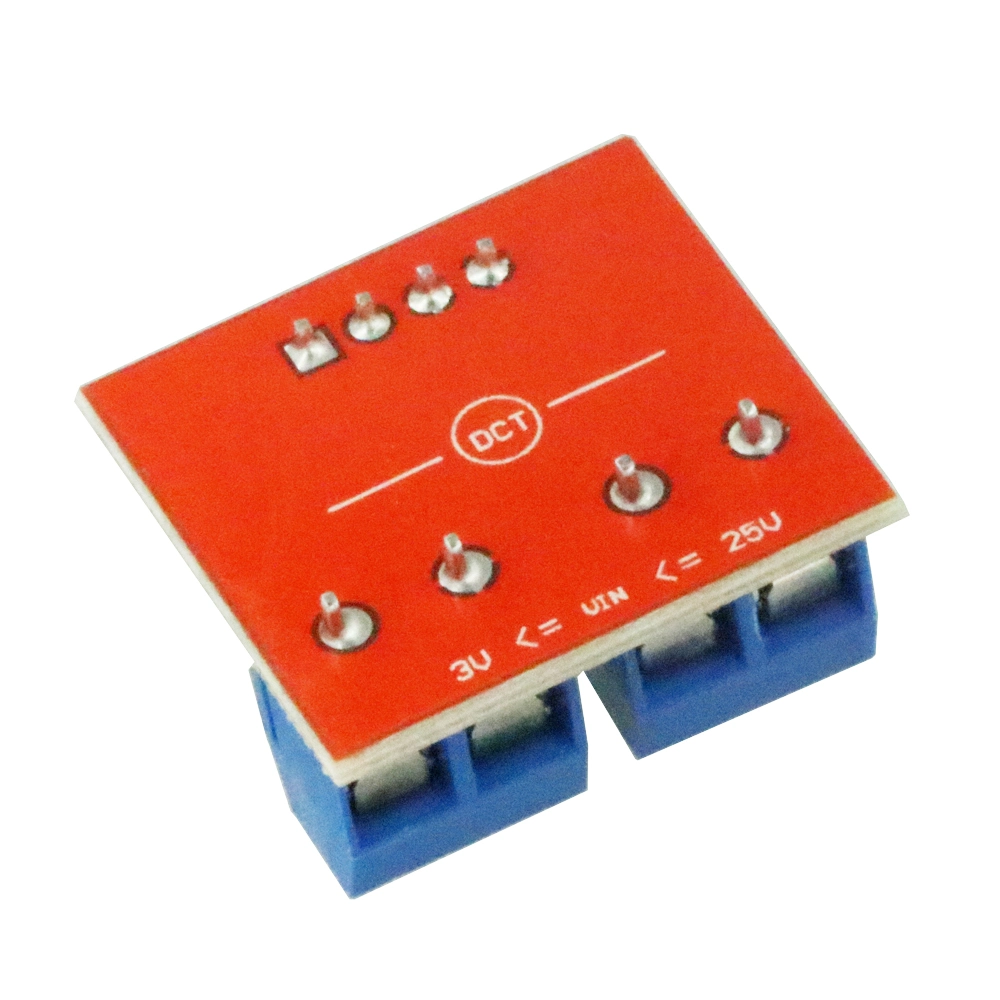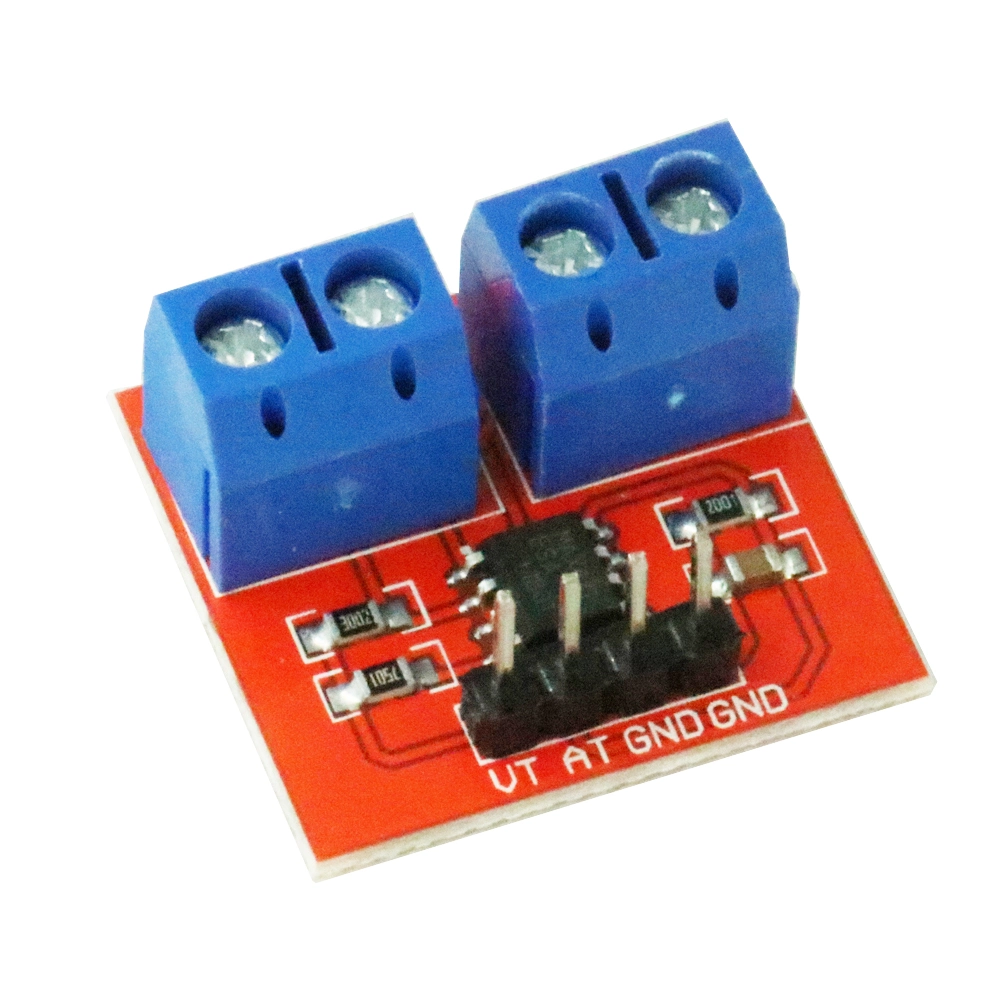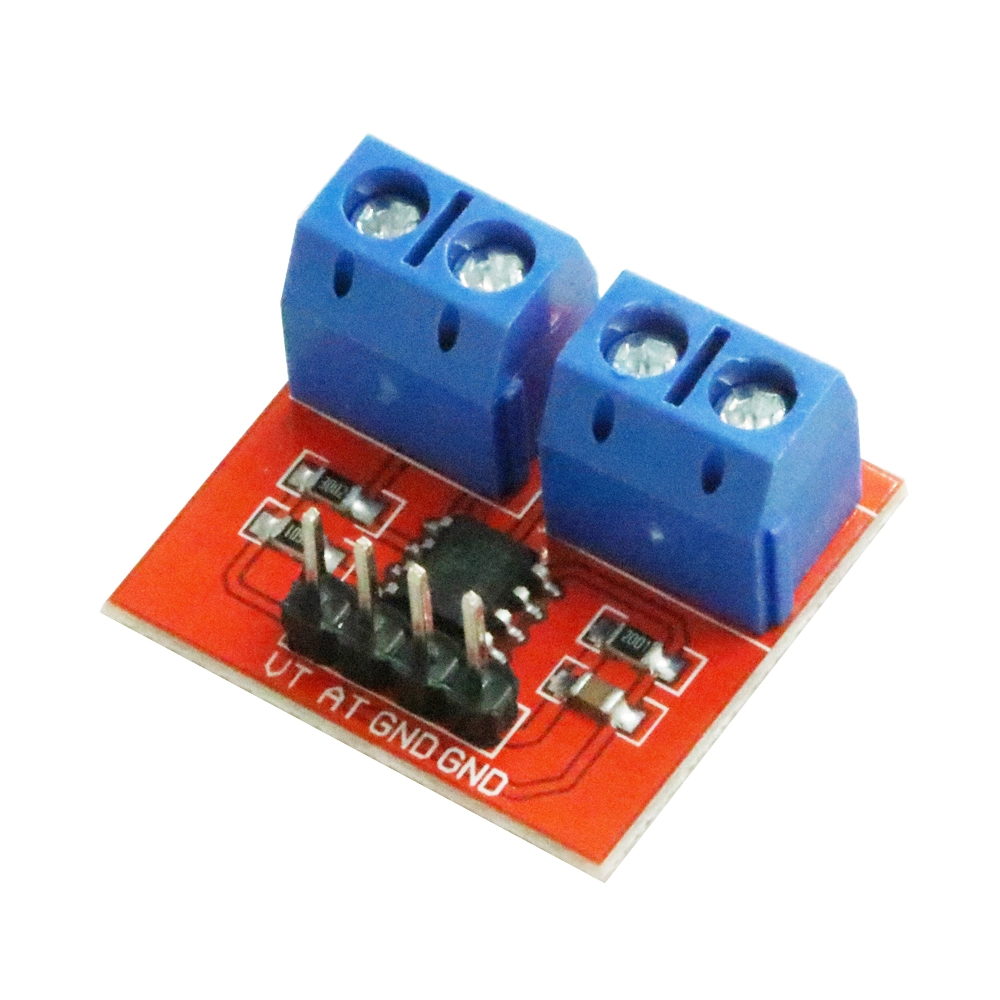# Max471 Voltage Current Sensor for Arduino

MAX471 Voltage Current Sensor for ArduinoThis module can be used to test the current and voltage.The principle of voltage test based on principle of resistive voltage divider design, can make the red terminal connector input voltage to 5 times smaller.Arduino analog input voltages up to 5 v, the voltage detection modul

# MAX471 Voltage Current Sensor for Arduino

This module can be used to test the current and voltage.
The principle of voltage test based on principle of resistive voltage divider design, can make the
red terminal connector input voltage to 5 times smaller.
the voltage detection module input voltage not greater than 5Vx5=25V (if using 3.3V systems, input voltage not greater than 3.3Vx5=16.5V).
Arduino AVR chips have 10-bit AD, so this module
simulates a resolution of 0.00489V (5V/1023), so the minimum voltage of input voltage detection module is 0.00489Vx5=0.02445V.

Features:
Test Voltage range: DC3 - 25V (Arduino work on 5V) or DC3 - 16.5V (Arduino work on 3.3V)
Test Current range: DC 0 - 3A

Example: (Working Voltage:5V)